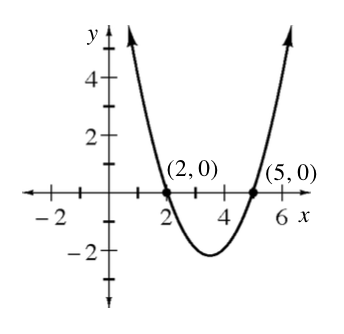### Home > INT2 > Chapter 9 > Lesson 9.4.1 > Problem9-134

9-134.This problem is a checkpoint for the quadratic web. It will be referred to as Checkpoint 9.

Determine the required missing part of the quadratic web.

1.Make an $x$ $y$ table and then draw a graph of $y = x^2 - 2x − 3$.

2. Write a possible equation for the given graph.

3. Without making an $x$ $y$ table, what are the $x$-intercepts of $y = x^2 + 8x + 7$? Use them to calculate the coordinates of the vertex and then sketch a graph.

4. The height (in feet) of a rocket after $x$ seconds is given by the equation $y = 128x - 16x^2$. What is the maximum height reached by the rocket and how long does it take to reach that height?

Check your answers by referring to the Checkpoint 9 materials located at the back of your book.

Ideally, at this point you are comfortable working with these types of problems and can solve them correctly. If you feel that you need more confidence when solving these types of problems, then review the Checkpoint 9 materials and try the practice problems provided. From this point on, you will be expected to do problems like these correctly and with confidence.

Answers and extra practice are located in the back of your printed textbook or in the Reference Tab of your eBook. If you have an eBook for INT2, login and then click the following link: Checkpoint 9: The Quadratic Web